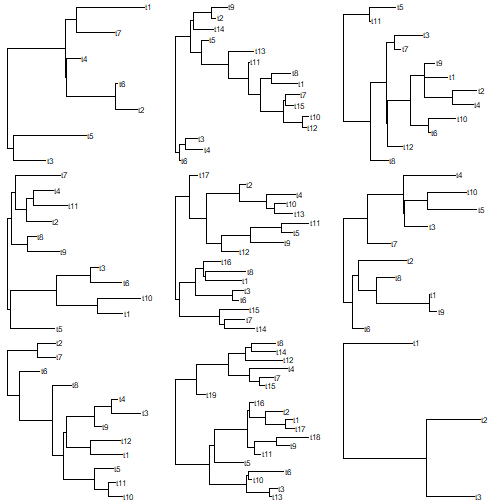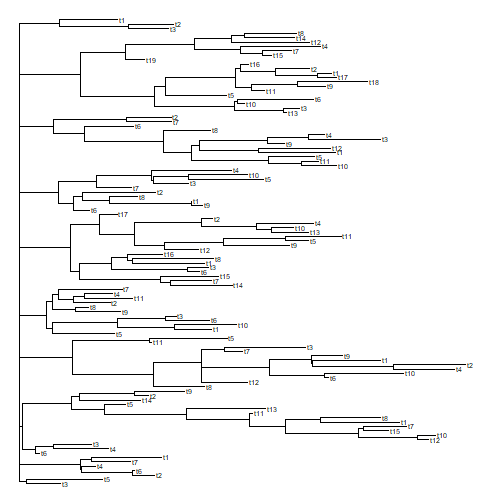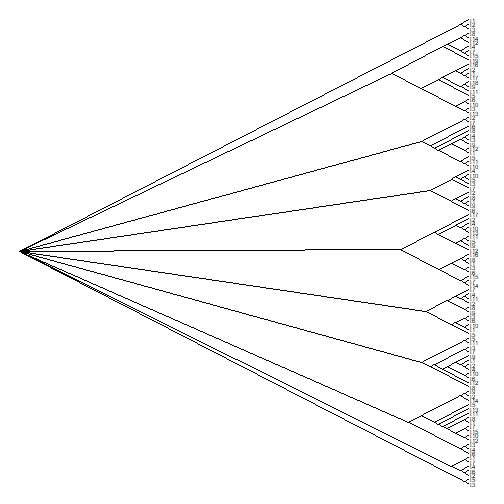## Tuesday, February 20, 2018

### Recursive `bind.tree` function

A phytools user contacted me today about coding a recursive version of `ape::bind.tree` - that is, a function that would take a list of trees & bind them successively together (based on his code, at the root) until only one tree remained.

A recursive function is nothing more than a function that calls itself internally. These are possible, though (in my experience) not that common, in R.

I'm not sure why we'd want to do this since a simple `for` or `while` loop could do the same thing, but I thought I'd help him out anyway.

Here's my recursive function:

``````bind.trees<-function(trees){
if(length(trees)==2) return(bind.tree(trees[],trees[]))
else {
trees<-c(bind.tree(trees[],trees[]),
if(length(trees)>2) trees[3:length(trees)])
trees<-bind.trees(trees) ## this is the recursive part
return(trees)
}
}
``````

We can test it out as follows:

``````trees<-lapply(sample(2:20,9,replace=TRUE),rtree)
trees<-lapply(trees,function(x){
x\$root.edge<-runif(n=1)
x})
class(trees)<-"multiPhylo"
print(trees,details=TRUE)
``````
``````## 9 phylogenetic trees
## tree 1 : 7 tips
## tree 2 : 15 tips
## tree 3 : 12 tips
## tree 4 : 11 tips
## tree 5 : 17 tips
## tree 6 : 10 tips
## tree 7 : 12 tips
## tree 8 : 19 tips
## tree 9 : 3 tips
``````
``````par(mfrow=c(3,3))
plotTree(trees,lwd=1)
````````````tree<-bind.trees(trees)
plotTree(tree,fsize=0.6,lwd=1)
``````Or, to better see the structure of all our original trees:

``````tree\$edge.length<-NULL